# RD Sharma Solutions For Class 7 Maths Exercise 8.4 Chapter 8 Linear Equations in One Variable

In order to excel in exams, students can refer and download the PDF of RD Sharma Solutions for Class 7 Maths Exercise 8.4 of Chapter 8 Linear Equation in One Variable are attached below. Students can easily get the PDF from given links. Our subject matter experts have solved these solutions on the basis of students exam point of view. This exercise discusses applications of linear equations to practical problems. This means these problems involve relations among unknown quantities and known quantities and often stated in words. Therefore it is referred to as word problems. Here word problem is first converted to the equation then we solve it by using any one of the methods. To know more about this, refer to RD Sharma Solutions for Class 7.

## Download the PDF of RD Sharma Solutions For Class 7 Maths Chapter 8 – Linear Equations in One variable Exercise 8.4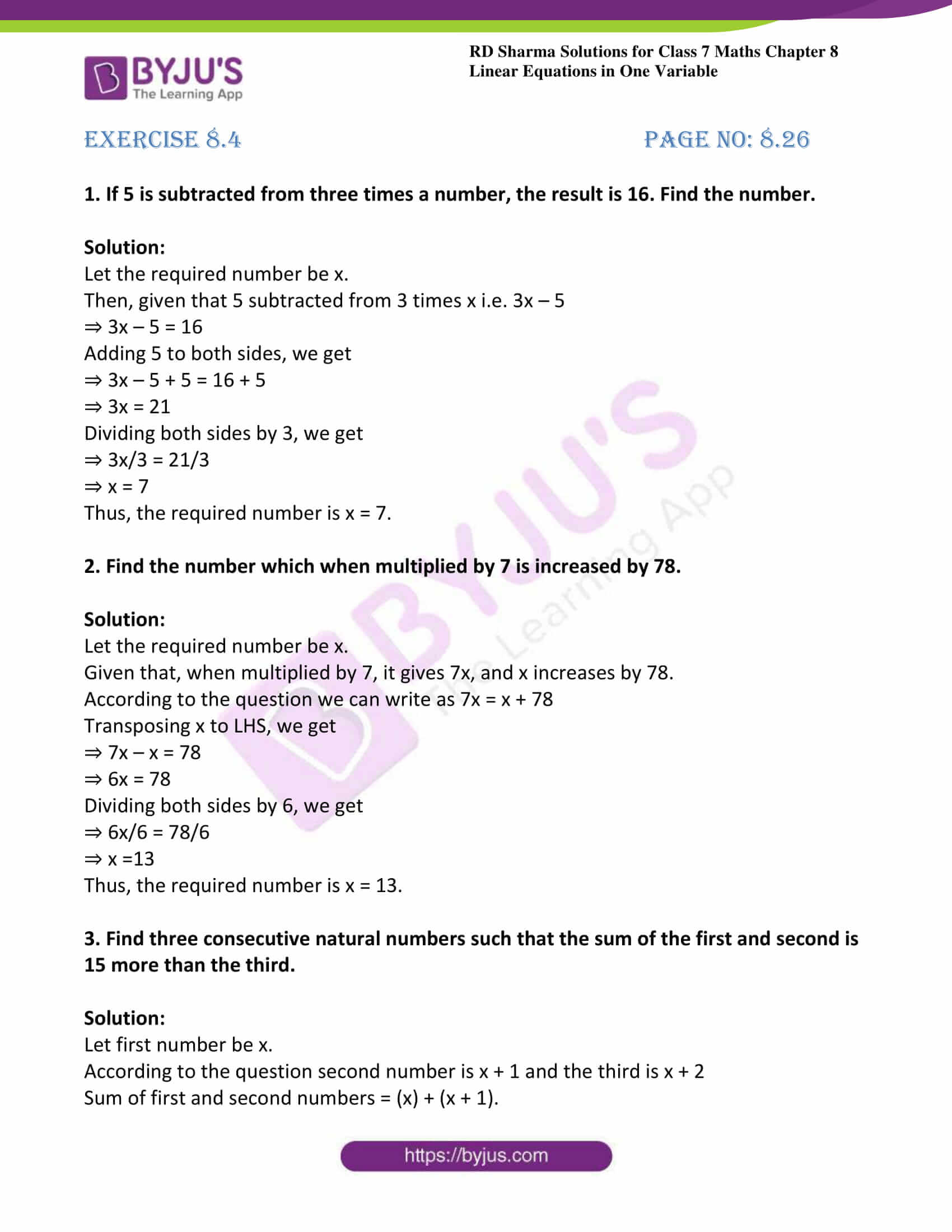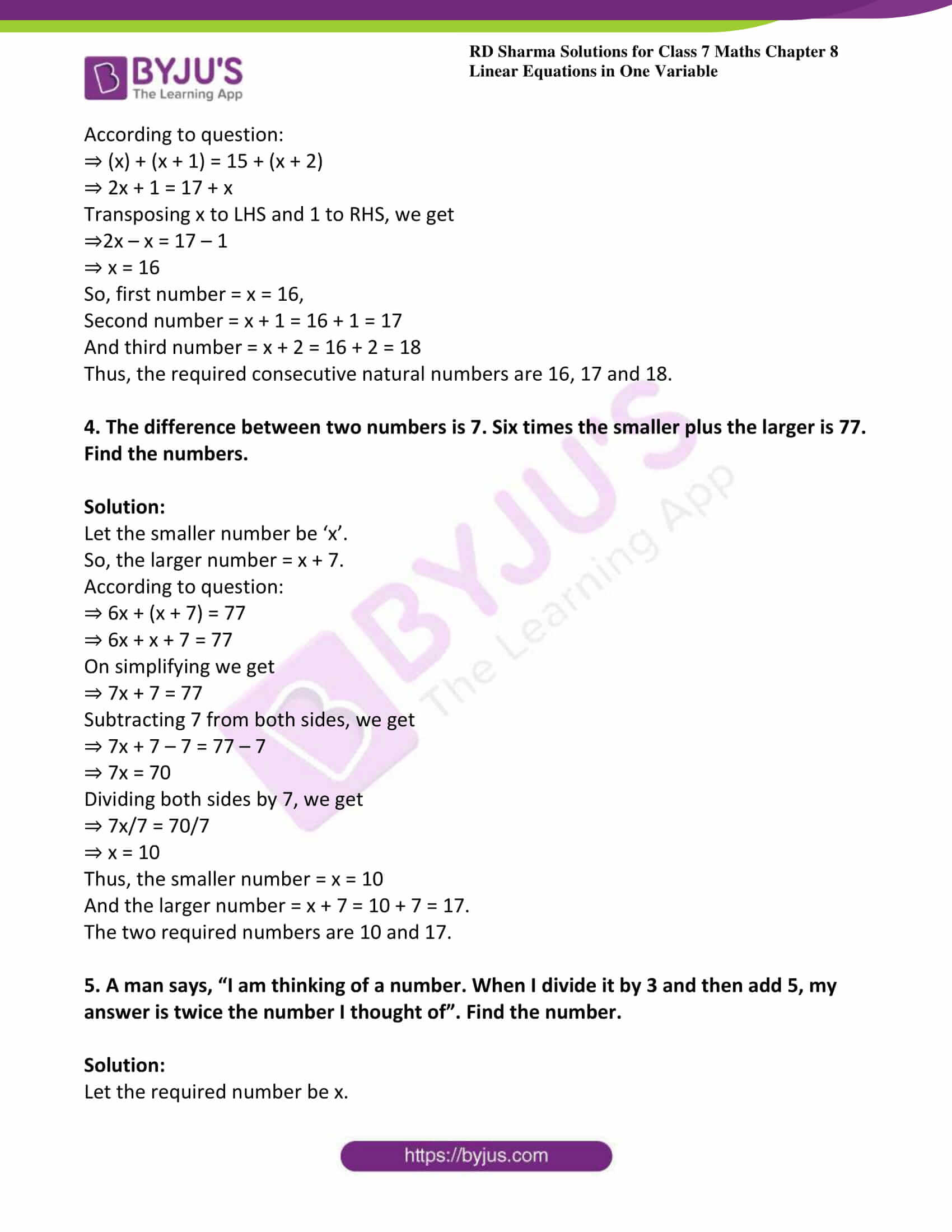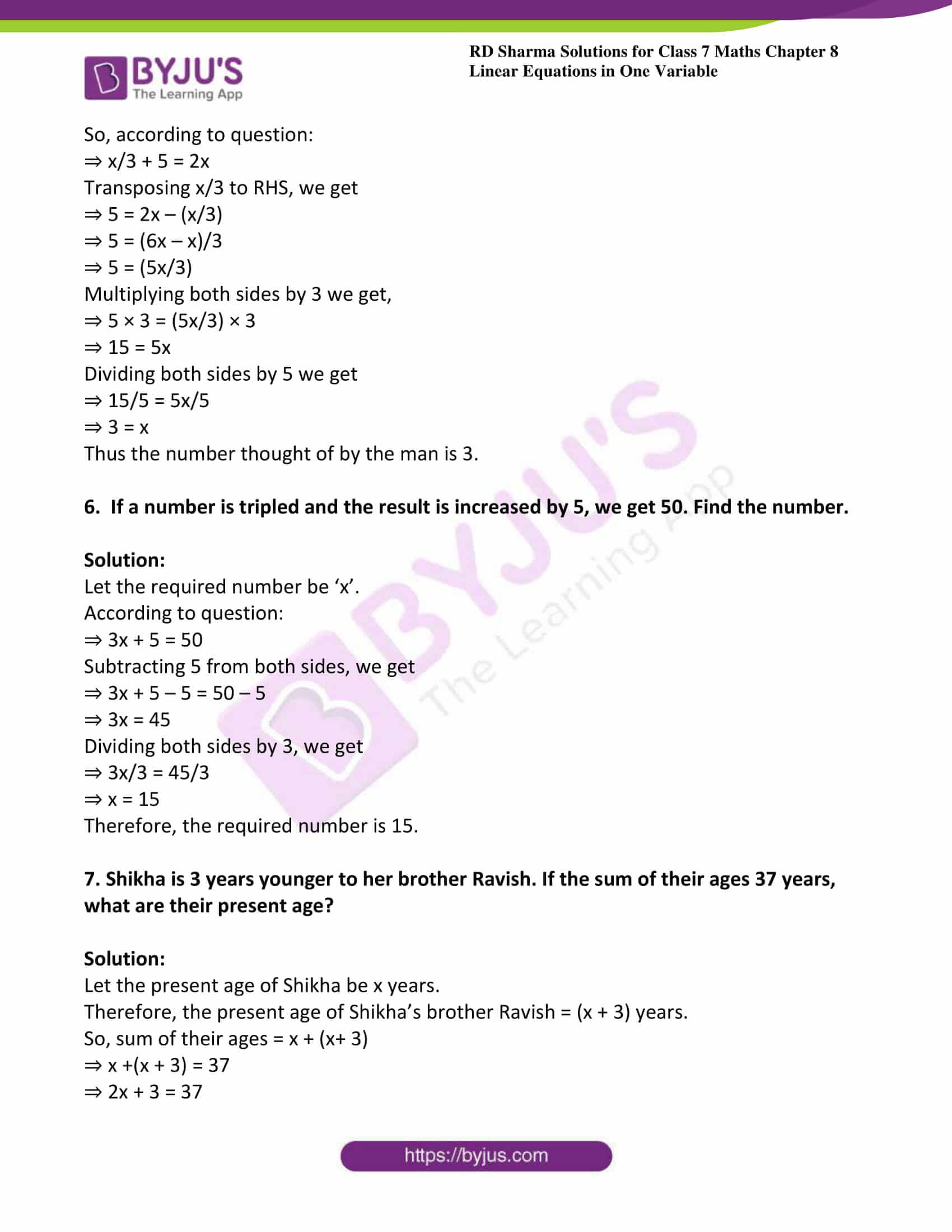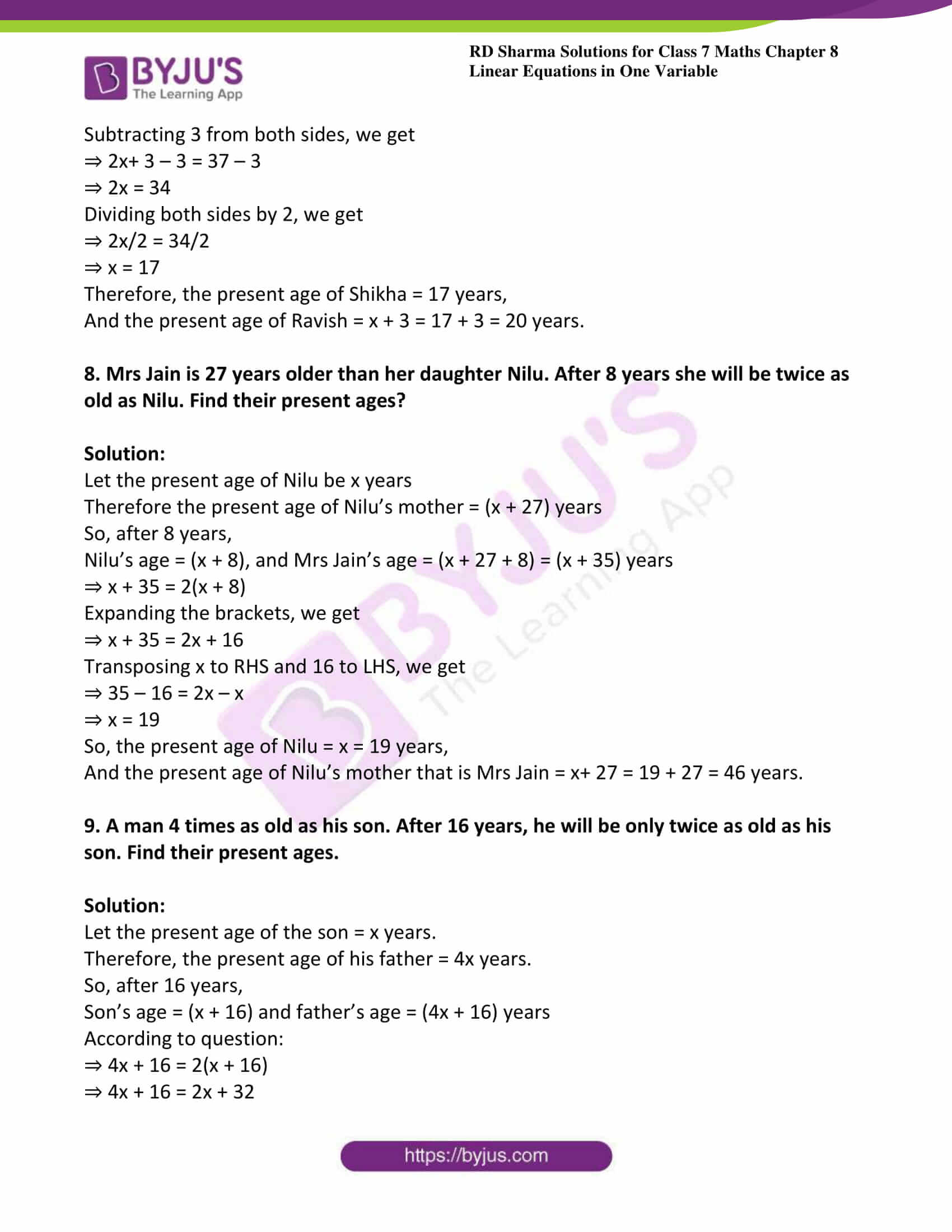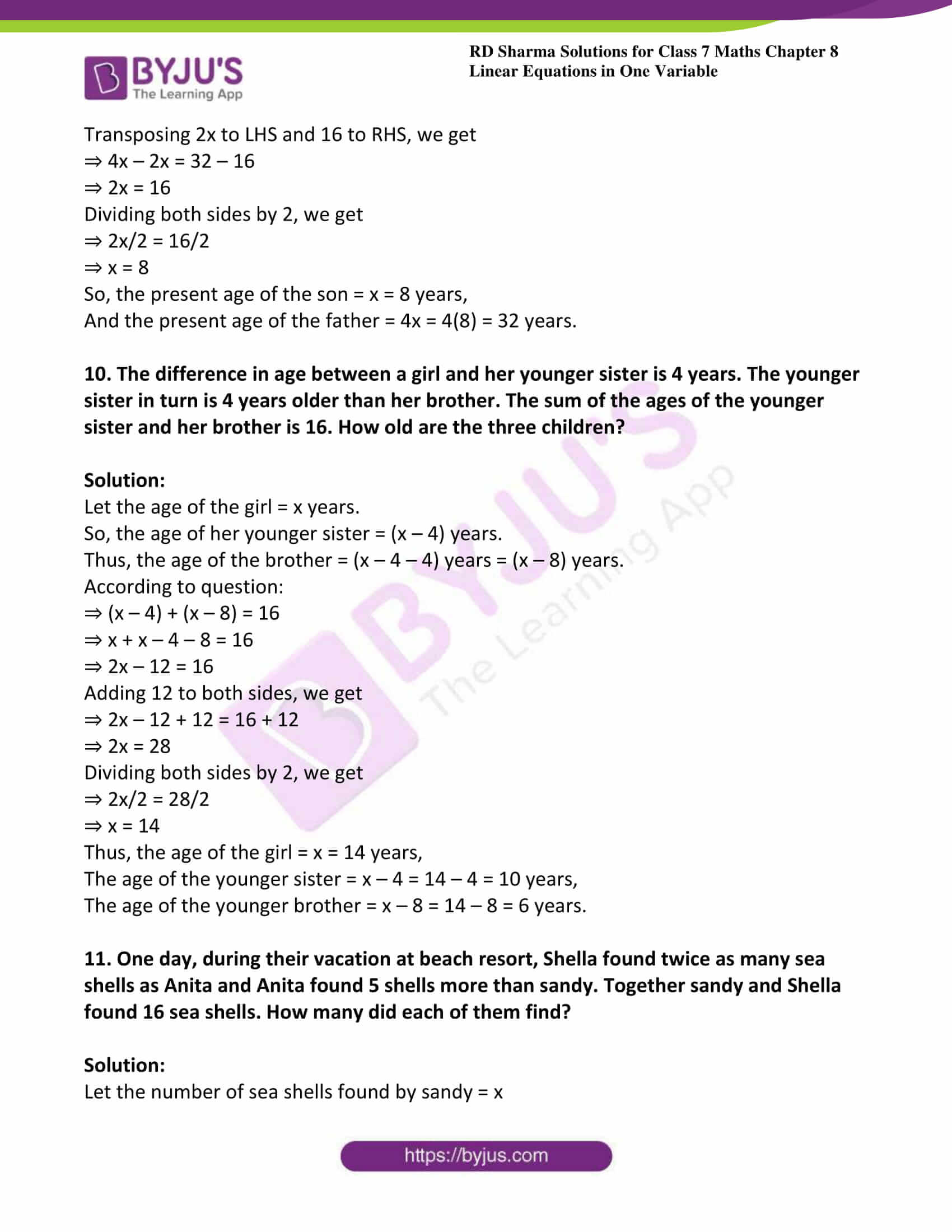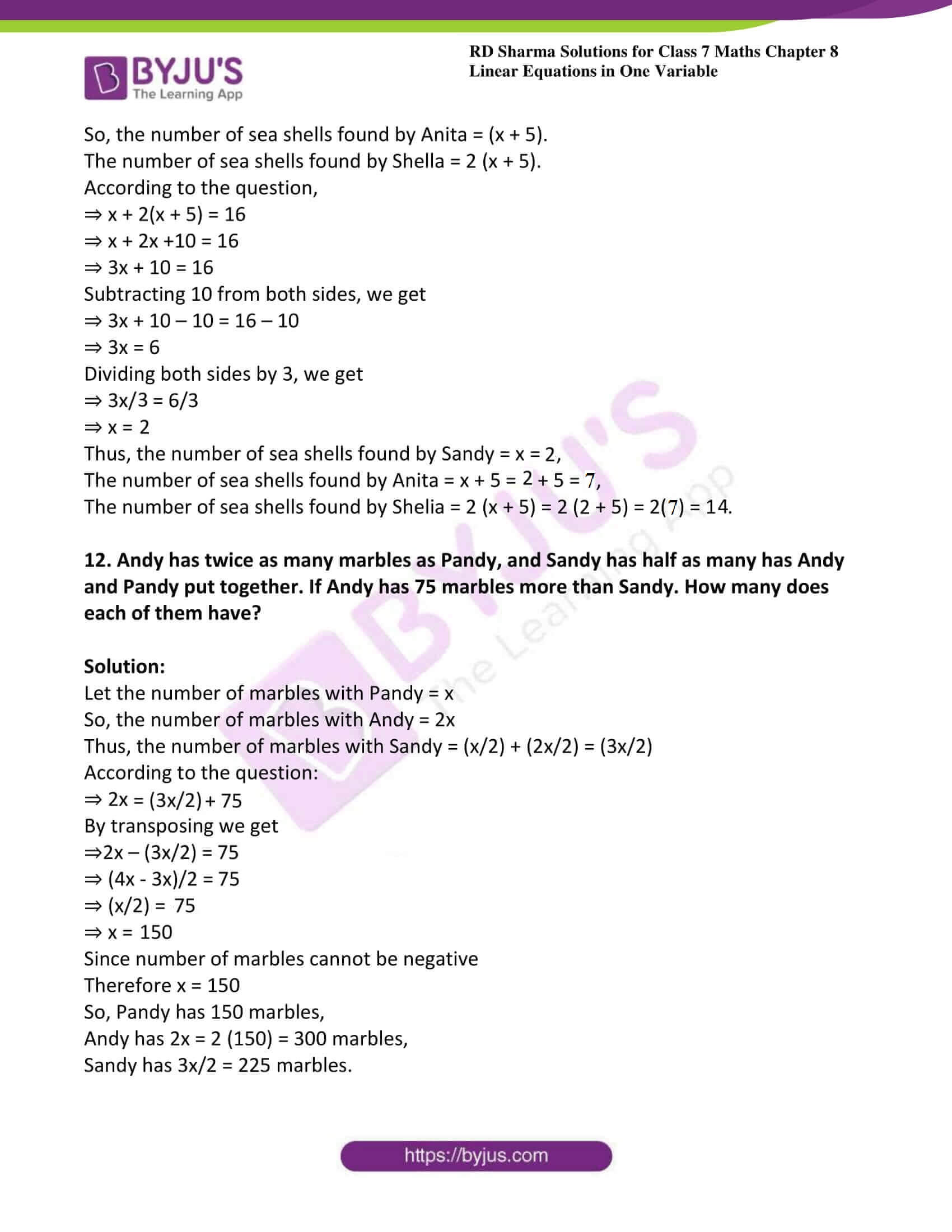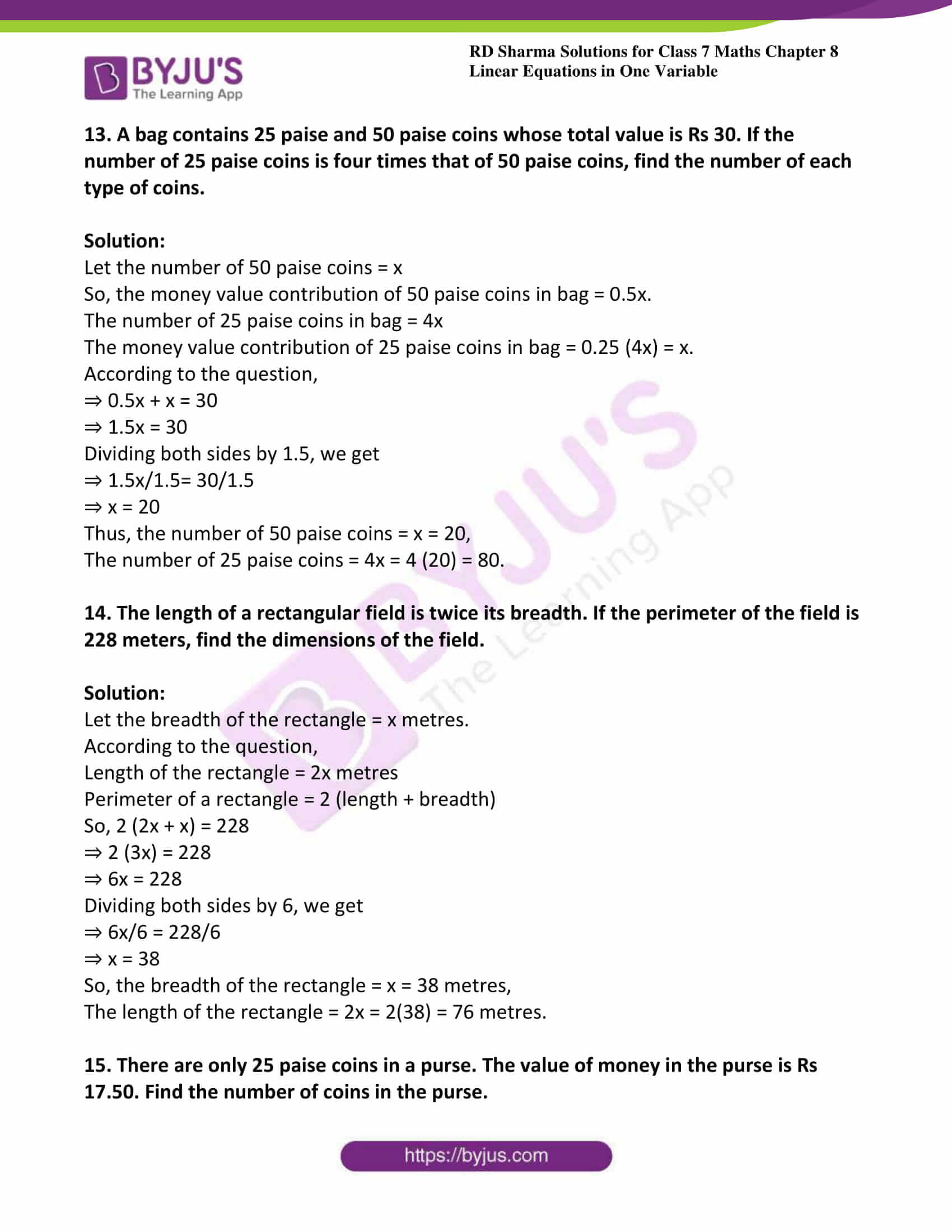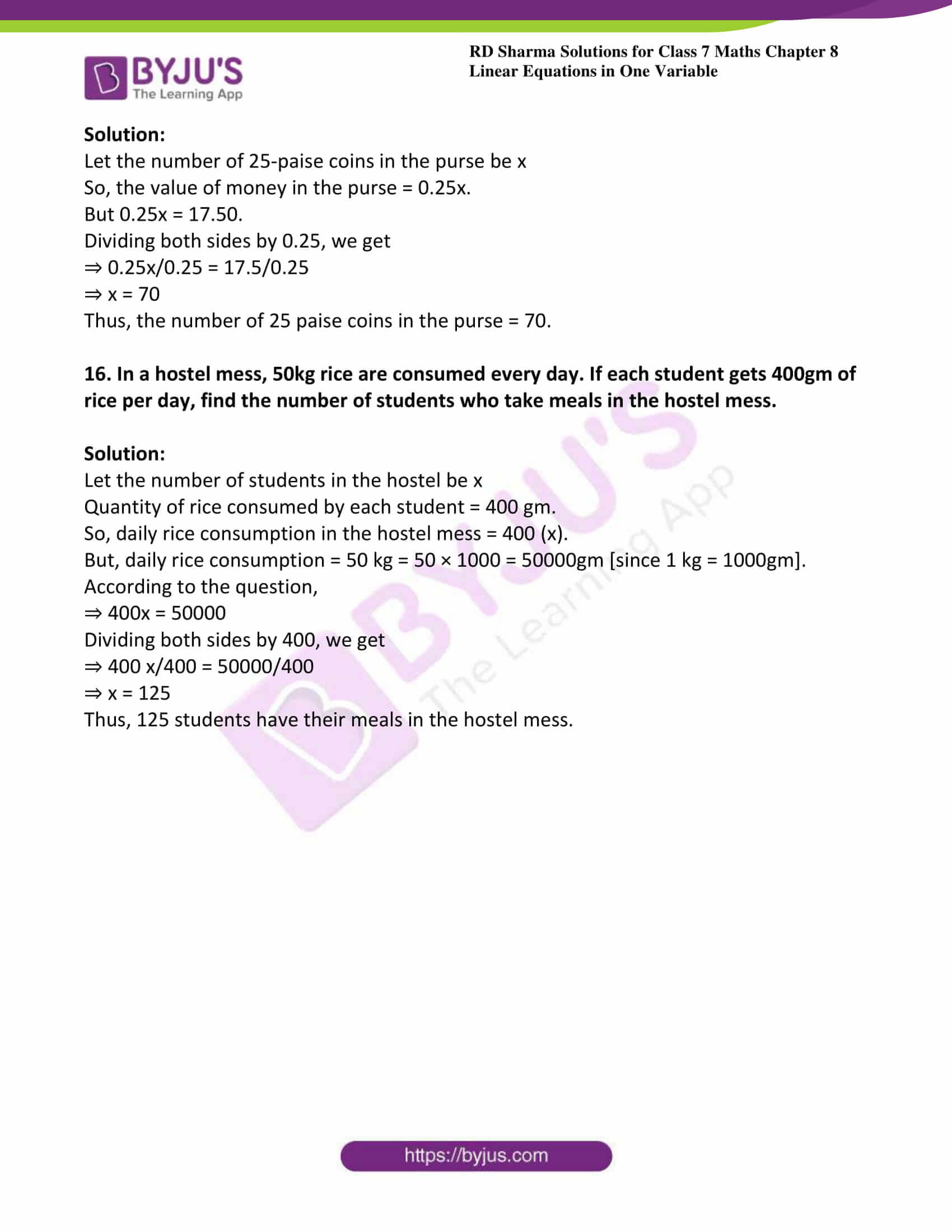### Access answers to Maths RD Sharma Solutions For Class 7 Chapter 8 – Linear Equation in One Variable Exercise 8.4

1. If 5 is subtracted from three times a number, the result is 16. Find the number.

Solution:

Let the required number be x.

Then, given that 5 subtracted from 3 times x i.e. 3x – 5

⇒ 3x – 5 = 16

Adding 5 to both sides, we get

⇒ 3x – 5 + 5 = 16 + 5

⇒ 3x = 21

Dividing both sides by 3, we get

⇒ 3x/3 = 21/3

⇒ x = 7

Thus, the required number is x = 7.

2. Find the number which when multiplied by 7 is increased by 78.

Solution:

Let the required number be x.

Given that, when multiplied by 7, it gives 7x, and x increases by 78.

According to the question we can write as 7x = x + 78

Transposing x to LHS, we get

⇒ 7x – x = 78

⇒ 6x = 78

Dividing both sides by 6, we get

⇒ 6x/6 = 78/6

⇒ x =13

Thus, the required number is x = 13.

3. Find three consecutive natural numbers such that the sum of the first and second is 15 more than the third.

Solution:

Let first number be x.

According to the question second number is x + 1 and the third is x + 2

Sum of first and second numbers = (x) + (x + 1).

According to question:

⇒ (x) + (x + 1) = 15 + (x + 2)

⇒ 2x + 1 = 17 + x

Transposing x to LHS and 1 to RHS, we get

⇒2x – x = 17 – 1

⇒ x = 16

So, first number = x = 16,

Second number = x + 1 = 16 + 1 = 17

And third number = x + 2 = 16 + 2 = 18

Thus, the required consecutive natural numbers are 16, 17 and 18.

4. The difference between two numbers is 7. Six times the smaller plus the larger is 77. Find the numbers.

Solution:

Let the smaller number be ‘x’.

So, the larger number = x + 7.

According to question:

⇒ 6x + (x + 7) = 77

⇒ 6x + x + 7 = 77

On simplifying we get

⇒ 7x + 7 = 77

Subtracting 7 from both sides, we get

⇒ 7x + 7 – 7 = 77 – 7

⇒ 7x = 70

Dividing both sides by 7, we get

⇒ 7x/7 = 70/7

⇒ x = 10

Thus, the smaller number = x = 10

And the larger number = x + 7 = 10 + 7 = 17.

The two required numbers are 10 and 17.

5. A man says, “I am thinking of a number. When I divide it by 3 and then add 5, my answer is twice the number I thought of”. Find the number.

Solution:

Let the required number be x.

So, according to question:

⇒ x/3 + 5 = 2x

Transposing x/3 to RHS, we get

⇒ 5 = 2x – (x/3)

⇒ 5 = (6x – x)/3

⇒ 5 = (5x/3)

Multiplying both sides by 3 we get,

⇒ 5 × 3 = (5x/3) × 3

⇒ 15 = 5x

Dividing both sides by 5 we get

⇒ 15/5 = 5x/5

⇒ 3 = x

Thus the number thought of by the man is 3.

6. If a number is tripled and the result is increased by 5, we get 50. Find the number.

Solution:

Let the required number be ‘x’.

According to question:

⇒ 3x + 5 = 50

Subtracting 5 from both sides, we get

⇒ 3x + 5 – 5 = 50 – 5

⇒ 3x = 45

Dividing both sides by 3, we get

⇒ 3x/3 = 45/3

⇒ x = 15

Therefore, the required number is 15.

7. Shikha is 3 years younger to her brother Ravish. If the sum of their ages 37 years, what are their present age?

Solution:

Let the present age of Shikha be x years.

Therefore, the present age of Shikha’s brother Ravish = (x + 3) years.

So, sum of their ages = x + (x+ 3)

⇒ x +(x + 3) = 37

⇒ 2x + 3 = 37

Subtracting 3 from both sides, we get

⇒ 2x+ 3 – 3 = 37 – 3

⇒ 2x = 34

Dividing both sides by 2, we get

⇒ 2x/2 = 34/2

⇒ x = 17

Therefore, the present age of Shikha = 17 years,

And the present age of Ravish = x + 3 = 17 + 3 = 20 years.

8. Mrs Jain is 27 years older than her daughter Nilu. After 8 years she will be twice as old as Nilu. Find their present ages?

Solution:

Let the present age of Nilu be x years

Therefore the present age of Nilu’s mother = (x + 27) years

So, after 8 years,

Nilu’s age = (x + 8), and Mrs Jain’s age = (x + 27 + 8) = (x + 35) years

⇒ x + 35 = 2(x + 8)

Expanding the brackets, we get

⇒ x + 35 = 2x + 16

Transposing x to RHS and 16 to LHS, we get

⇒ 35 – 16 = 2x – x

⇒ x = 19

So, the present age of Nilu = x = 19 years,

And the present age of Nilu’s mother that is Mrs Jain = x+ 27 = 19 + 27 = 46 years.

9. A man 4 times as old as his son. After 16 years, he will be only twice as old as his son. Find their present ages.

Solution:

Let the present age of the son = x years.

Therefore, the present age of his father = 4x years.

So, after 16 years,

Son’s age = (x + 16) and father’s age = (4x + 16) years

According to question:

⇒ 4x + 16 = 2(x + 16)

⇒ 4x + 16 = 2x + 32

Transposing 2x to LHS and 16 to RHS, we get

⇒ 4x – 2x = 32 – 16

⇒ 2x = 16

Dividing both sides by 2, we get

⇒ 2x/2 = 16/2

⇒ x = 8

So, the present age of the son = x = 8 years,

And the present age of the father = 4x = 4(8) = 32 years.

10. The difference in age between a girl and her younger sister is 4 years. The younger sister in turn is 4 years older than her brother. The sum of the ages of the younger sister and her brother is 16. How old are the three children?

Solution:

Let the age of the girl = x years.

So, the age of her younger sister = (x – 4) years.

Thus, the age of the brother = (x – 4 – 4) years = (x – 8) years.

According to question:

⇒ (x – 4) + (x – 8) = 16

⇒ x + x – 4 – 8 = 16

⇒ 2x – 12 = 16

Adding 12 to both sides, we get

⇒ 2x – 12 + 12 = 16 + 12

⇒ 2x = 28

Dividing both sides by 2, we get

⇒ 2x/2 = 28/2

⇒ x = 14

Thus, the age of the girl = x = 14 years,

The age of the younger sister = x – 4 = 14 – 4 = 10 years,

The age of the younger brother = x – 8 = 14 – 8 = 6 years.

11. One day, during their vacation at beach resort, Shella found twice as many sea shells as Anita and Anita found 5 shells more than sandy. Together sandy and Shella found 16 sea shells. How many did each of them find?

Solution:

Let the number of sea shells found by sandy = x

So, the number of sea shells found by Anita = (x + 5).

The number of sea shells found by Shella = 2 (x + 5).

According to the question,

⇒ x + 2(x + 5) = 16

⇒ x + 2x +10 = 16

⇒ 3x + 10 = 16

Subtracting 10 from both sides, we get

⇒ 3x + 10 – 10 = 16 – 10

⇒ 3x = 6

Dividing both sides by 3, we get

⇒ 3x/3 = 6/3

⇒ x = 2

Thus, the number of sea shells found by Sandy = x = 2,

The number of sea shells found by Anita = x + 5 = 2 + 5 = 7,

The number of sea shells found by Shelia = 2 (x + 5) = 2 (2 + 5) = 2(7) = 14.

12. Andy has twice as many marbles as Pandy, and Sandy has half as many has Andy and Pandy put together. If Andy has 75 marbles more than Sandy. How many does each of them have?

Solution:

Let the number of marbles with Pandy = x

So, the number of marbles with Andy = 2x

Thus, the number of marbles with Sandy = (x/2) + (2x/2) = (3x/2)

According to the question:

⇒ 2x = (3x/2) + 75

By transposing we get

⇒2x – (3x/2) = 75

⇒ (4x – 3x)/2 = 75

⇒ (x/2) = 75

⇒ x = 150

Since number of marbles cannot be negative

Therefore x = 150

So, Pandy has 150 marbles,

Andy has 2x = 2 (150) = 300 marbles,

Sandy has 3x/2 = 225 marbles.

13. A bag contains 25 paise and 50 paise coins whose total value is Rs 30. If the number of 25 paise coins is four times that of 50 paise coins, find the number of each type of coins.

Solution:

Let the number of 50 paise coins = x

So, the money value contribution of 50 paise coins in bag = 0.5x.

The number of 25 paise coins in bag = 4x

The money value contribution of 25 paise coins in bag = 0.25 (4x) = x.

According to the question,

⇒ 0.5x + x = 30

⇒ 1.5x = 30

Dividing both sides by 1.5, we get

⇒ 1.5x/1.5= 30/1.5

⇒ x = 20

Thus, the number of 50 paise coins = x = 20,

The number of 25 paise coins = 4x = 4 (20) = 80.

14. The length of a rectangular field is twice its breadth. If the perimeter of the field is 228 meters, find the dimensions of the field.

Solution:

Let the breadth of the rectangle = x metres.

According to the question,

Length of the rectangle = 2x metres

Perimeter of a rectangle = 2 (length + breadth)

So, 2 (2x + x) = 228

⇒ 2 (3x) = 228

⇒ 6x = 228

Dividing both sides by 6, we get

⇒ 6x/6 = 228/6

⇒ x = 38

So, the breadth of the rectangle = x = 38 metres,

The length of the rectangle = 2x = 2(38) = 76 metres.

15. There are only 25 paise coins in a purse. The value of money in the purse is Rs 17.50. Find the number of coins in the purse.

Solution:

Let the number of 25-paise coins in the purse be x

So, the value of money in the purse = 0.25x.

But 0.25x = 17.50.

Dividing both sides by 0.25, we get

⇒ 0.25x/0.25 = 17.5/0.25

⇒ x = 70

Thus, the number of 25 paise coins in the purse = 70.

16. In a hostel mess, 50kg rice are consumed every day. If each student gets 400gm of rice per day, find the number of students who take meals in the hostel mess.

Solution:

Let the number of students in the hostel be x

Quantity of rice consumed by each student = 400 gm.

So, daily rice consumption in the hostel mess = 400 (x).

But, daily rice consumption = 50 kg = 50 × 1000 = 50000gm [since 1 kg = 1000gm].

According to the question,

⇒ 400x = 50000

Dividing both sides by 400, we get

⇒ 400 x/400 = 50000/400

⇒ x = 125

Thus, 125 students have their meals in the hostel mess.